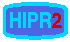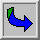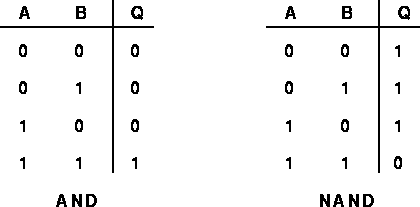# Logical Operators

Logical operators are generally derived from Boolean algebra, which is a mathematical way of manipulating the truth values of concepts in an abstract way without bothering about what the concepts actually mean. The truth value of a concept in Boolean value can have just one of two possible values: true or false. Boolean algebra allows you to represent things like:

The block is both red and large

by something like:

A AND B

where A represents `The block is red', and B represents `The block is large'. Now each of these sub-phrases has its own truth value in any given situation: each sub-phrase is either true or false. Moreover, the entire composite phrase also has a truth value: it is true if both of the sub-phrases are true, and false in any other case. We can write this AND combination rule (and its dual operation NAND) using a truth-table as shown in Figure 1, in which we conventionally represent true by 1, and false by zero.Figure 1 Truth-tables for AND and NAND

The left hand table shows each of the possible combinations of truth values of A and B, and the the resulting truth value of A AND B. Similar truth-tables can be set up for the other logical operators: NAND, OR, NOR, XOR, XNOR and NOT.

Turning now to an image processing context, the pixel values in a binary image, which are either 0 or 1, can be interpreted as truth values as above. Using this convention we can carry out logical operations on images simply by applying the truth-table combination rules to the pixel values from a pair of input images (or a single input image in the case of NOT). Normally, corresponding pixels from each of two identically sized binary input images are compared to produce the output image, which is another binary image of the same size. As with other image arithmetic operations, it is also possible to logically combine a single input image with a constant logical value, in which case each pixel in the input image is compared to the same constant in order to produce the corresponding output pixel. See the individual logical operator descriptions for examples of these operations.Logical operations can also be carried out on images with integer pixel values. In this extension the logical operations are normally carried out in bitwise fashion on binary representations of those integers, comparing corresponding bits with corresponding bits to produce the output pixel value. For instance, suppose that we wish to XOR the integers 47 and 255 together using 8-bit integers. 47 is 00101111 in binary and 255 is 11111111. XORing these together in bitwise fashion, we have 11010000 in binary or 208 in decimal.

Note that not all implementations of logical operators work in such bitwise fashion. For instance some will treat zero as false and any non-zero value as true and will then apply the conventional 1-bit logical functions to derive the output image. The output may be a simple binary image itself, or it may be a graylevel image formed perhaps by multiplying what would be the binary output image (containing 0's and 1's) with one of the input images.